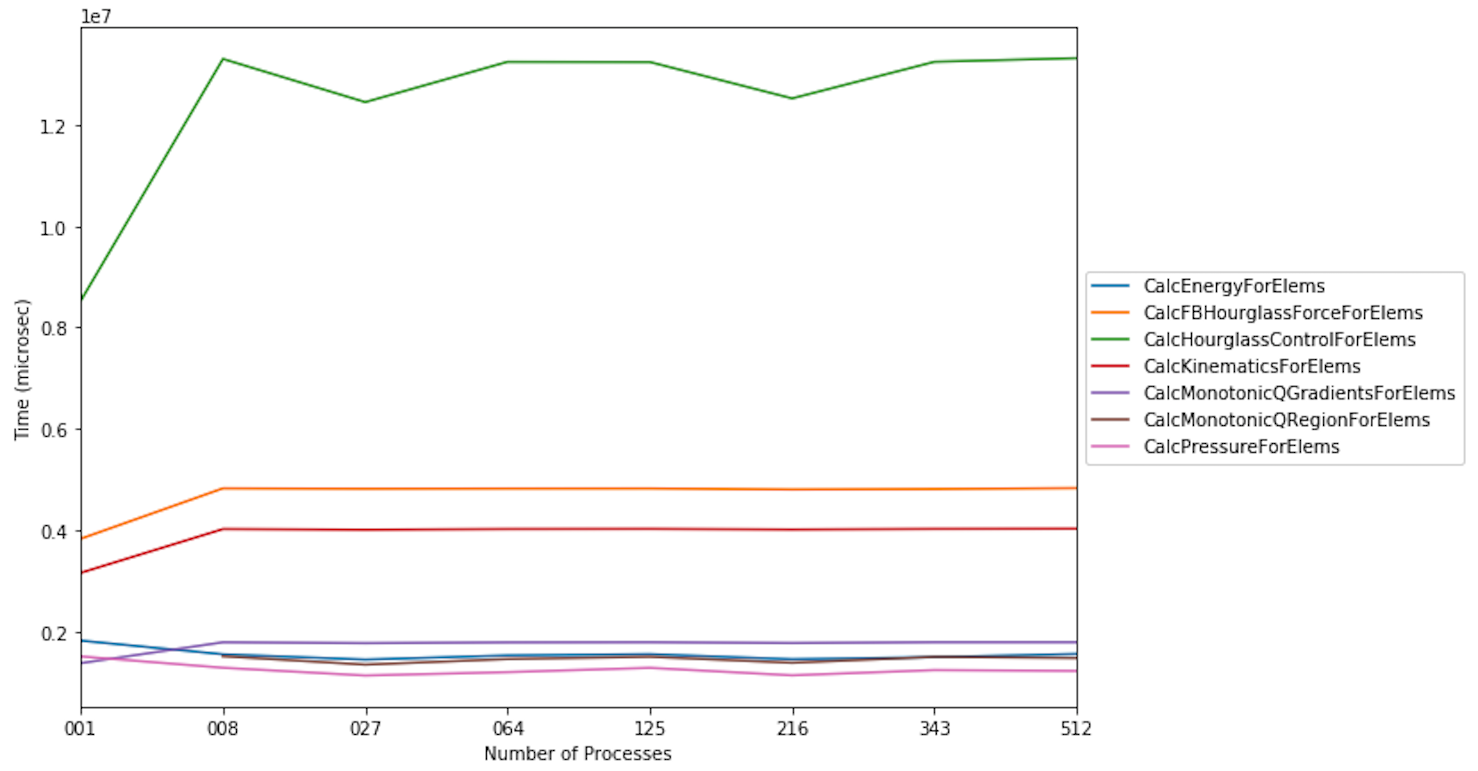# Analysis Examples¶

## Reading different file formats¶

Hatchet can read in a variety of data file formats into a GraphFrame. Below, we show examples of reading in different data formats.

### Read in an HPCToolkit database¶

A database directory is generated by using `hpcprof-mpi` to post-process the raw measurements directory output by HPCToolkit. To analyze an HPCToolkit database, the `from_hpctoolkit` method can be used.

```#!/usr/bin/env python

import hatchet as ht

if __name__ == "__main__":
# Path to HPCToolkit database directory.
dirname = "../../../hatchet/tests/data/hpctoolkit-cpi-database"

# Use hatchet's ``from_hpctoolkit`` API to read in the HPCToolkit database.
# The result is stored into Hatchet's GraphFrame.
gf = ht.GraphFrame.from_hpctoolkit(dirname)

# Printout the DataFrame component of the GraphFrame.
print(gf.dataframe)

# Printout the graph component of the GraphFrame.
# Use "time (inc)" as the metric column to be displayed
print(gf.tree(metric_column="time (inc)"))
```

### Read in a Caliper cali file¶

Caliper’s default raw performance data output is the cali. The cali format can be read by `cali-query`, which transforms the raw data into JSON format.

```#!/usr/bin/env python

import hatchet as ht

if __name__ == "__main__":
# Path to caliper cali file.
cali_file = (
"../../../hatchet/tests/data/caliper-lulesh-cali/lulesh-annotation-profile.cali"
)

# Setup desired cali query.
grouping_attribute = "function"
default_metric = "sum(sum#time.duration),inclusive_sum(sum#time.duration)"
query = "select function,%s group by %s format json-split" % (
default_metric,
grouping_attribute,
)

# Use hatchet's ``from_caliper`` API with the path to the cali file and the
# query. This API will internally run ``cali-query`` on this file to
# produce a json-split stream. The result is stored into Hatchet's
# GraphFrame.
gf = ht.GraphFrame.from_caliper(cali_file, query)

# Printout the DataFrame component of the GraphFrame.
print(gf.dataframe)

# Printout the graph component of the GraphFrame.
# Use "time (inc)" as the metric column to be displayed
print(gf.tree(metric_column="time (inc)"))
```

### Read in a Caliper JSON stream or file¶

Caliper’s json-split format writes a JSON file with separate fields for Caliper records and metadata. The json-split format is generated by either running `cali-query` on the raw Caliper data or by enabling the `mpireport` service when using Caliper.

#### JSON Stream¶

```#!/usr/bin/env python

import subprocess
import hatchet as ht

if __name__ == "__main__":
# Path to caliper cali file.
cali_file = (
"../../../hatchet/tests/data/caliper-lulesh-cali/lulesh-annotation-profile.cali"
)

# Setup desired cali query.
cali_query = "cali-query"
grouping_attribute = "function"
default_metric = "sum(sum#time.duration),inclusive_sum(sum#time.duration)"
query = "select function,%s group by %s format json-split" % (
default_metric,
grouping_attribute,
)

# Use ``cali-query`` here to produce the json-split stream.
cali_json = subprocess.Popen(
[cali_query, "-q", query, cali_file], stdout=subprocess.PIPE
)

# Use hatchet's ``from_caliper_json`` API with the resulting json-split.
# The result is stored into Hatchet's GraphFrame.
gf = ht.GraphFrame.from_caliper_json(cali_json.stdout)

# Printout the DataFrame component of the GraphFrame.
print(gf.dataframe)

# Printout the graph component of the GraphFrame.
# Use "time (inc)" as the metric column to be displayed
print(gf.tree(metric_column="time (inc)"))
```

#### JSON File¶

```#!/usr/bin/env python

import hatchet as ht

if __name__ == "__main__":
# Path to caliper json-split file.
json_file = "../../../hatchet/tests/data/caliper-cpi-json/cpi-callpath-profile.json"

# Use hatchet's ``from_caliper_json`` API with the resulting json-split.
# The result is stored into Hatchet's GraphFrame.
gf = ht.GraphFrame.from_caliper_json(json_file)

# Printout the DataFrame component of the GraphFrame.
print(gf.dataframe)

# Printout the graph component of the GraphFrame.
# Because no metric parameter is specified, ``time`` is used by default.
print(gf.tree())
```

### Read in a DOT file¶

The DOT file format is generated by using `gprof2dot` on `gprof` or `callgrind` output.

```#!/usr/bin/env python

import hatchet as ht

if __name__ == "__main__":
# Path to DOT file.
dot_file = "../../../hatchet/tests/data/gprof2dot-cpi/callgrind.dot.64042.0.1"

# Use hatchet's ``from_gprof_dot`` API to read in the DOT file. The result
# is stored into Hatchet's GraphFrame.
gf = ht.GraphFrame.from_gprof_dot(dot_file)

# Printout the DataFrame component of the GraphFrame.
print(gf.dataframe)

# Printout the graph component of the GraphFrame.
# Because no metric parameter is specified, ``time`` is used by default.
print(gf.tree())
```

### Read in a DAG literal¶

The literal format is a list of dictionaries representing a graph with nodes and metrics.

```#!/usr/bin/env python
# -*- encoding: utf-8 -*-

import hatchet as ht

if __name__ == "__main__":
# Define a literal GraphFrame using a list of dicts.
gf = ht.GraphFrame.from_literal(
[
{
"frame": {"name": "foo"},
"metrics": {"time (inc)": 130.0, "time": 0.0},
"children": [
{
"frame": {"name": "bar"},
"metrics": {"time (inc)": 20.0, "time": 5.0},
"children": [
{
"frame": {"name": "baz"},
"metrics": {"time (inc)": 5.0, "time": 5.0},
},
{
"frame": {"name": "grault"},
"metrics": {"time (inc)": 10.0, "time": 10.0},
},
],
},
{
"frame": {"name": "qux"},
"metrics": {"time (inc)": 60.0, "time": 0.0},
"children": [
{
"frame": {"name": "quux"},
"metrics": {"time (inc)": 60.0, "time": 5.0},
"children": [
{
"frame": {"name": "corge"},
"metrics": {"time (inc)": 55.0, "time": 10.0},
"children": [
{
"frame": {"name": "bar"},
"metrics": {
"time (inc)": 20.0,
"time": 5.0,
},
"children": [
{
"frame": {"name": "baz"},
"metrics": {
"time (inc)": 5.0,
"time": 5.0,
},
},
{
"frame": {"name": "grault"},
"metrics": {
"time (inc)": 10.0,
"time": 10.0,
},
},
],
},
{
"frame": {"name": "grault"},
"metrics": {
"time (inc)": 10.0,
"time": 10.0,
},
},
{
"frame": {"name": "garply"},
"metrics": {
"time (inc)": 15.0,
"time": 15.0,
},
},
],
}
],
}
],
},
{
"frame": {"name": "waldo"},
"metrics": {"time (inc)": 50.0, "time": 0.0},
"children": [
{
"frame": {"name": "fred"},
"metrics": {"time (inc)": 35.0, "time": 5.0},
"children": [
{
"frame": {"name": "plugh"},
"metrics": {"time (inc)": 5.0, "time": 5.0},
},
{
"frame": {"name": "xyzzy"},
"metrics": {"time (inc)": 25.0, "time": 5.0},
"children": [
{
"frame": {"name": "thud"},
"metrics": {
"time (inc)": 25.0,
"time": 5.0,
},
"children": [
{
"frame": {"name": "baz"},
"metrics": {
"time (inc)": 5.0,
"time": 5.0,
},
},
{
"frame": {"name": "garply"},
"metrics": {
"time (inc)": 15.0,
"time": 15.0,
},
},
],
}
],
},
],
},
{
"frame": {"name": "garply"},
"metrics": {"time (inc)": 15.0, "time": 15.0},
},
],
},
],
},
{
"frame": {"name": "ほげ (hoge)"},
"metrics": {"time (inc)": 30.0, "time": 0.0},
"children": [
{
"frame": {"name": "(ぴよ (piyo)"},
"metrics": {"time (inc)": 15.0, "time": 5.0},
"children": [
{
"frame": {"name": "ふが (fuga)"},
"metrics": {"time (inc)": 5.0, "time": 5.0},
},
{
"frame": {"name": "ほげら (hogera)"},
"metrics": {"time (inc)": 5.0, "time": 5.0},
},
],
},
{
"frame": {"name": "ほげほげ (hogehoge)"},
"metrics": {"time (inc)": 15.0, "time": 15.0},
},
],
},
]
)

# Printout the DataFrame component of the GraphFrame.
print(gf.dataframe)

# Printout the graph component of the GraphFrame.
# Because no metric parameter is specified, ``time`` is used by default.
print(gf.tree(metric_column=["time (inc)", "time"]))
```

## Basic Examples¶

### Applying scalar operations to attributes¶

Individual numeric columns in the dataframe can be scaled or offset by a constant using the native pandas operations. We make a copy of the original graphframe, and modify the dataframe directly. In this example, we offset the `time` column by -2 and scale it by 1/1e7, storing the result in a new column in the dataframe called `scaled time`.

```gf = ht.GraphFrame.from_hpctoolkit('kripke')
gf.drop_index_levels()

offset = 1e7
gf.dataframe['scaled time'] = (gf.dataframe['time'] / offset) - 2
sorted_df = gf.dataframe.sort_values(by=['scaled time'], ascending=False)
print(sorted_df)
```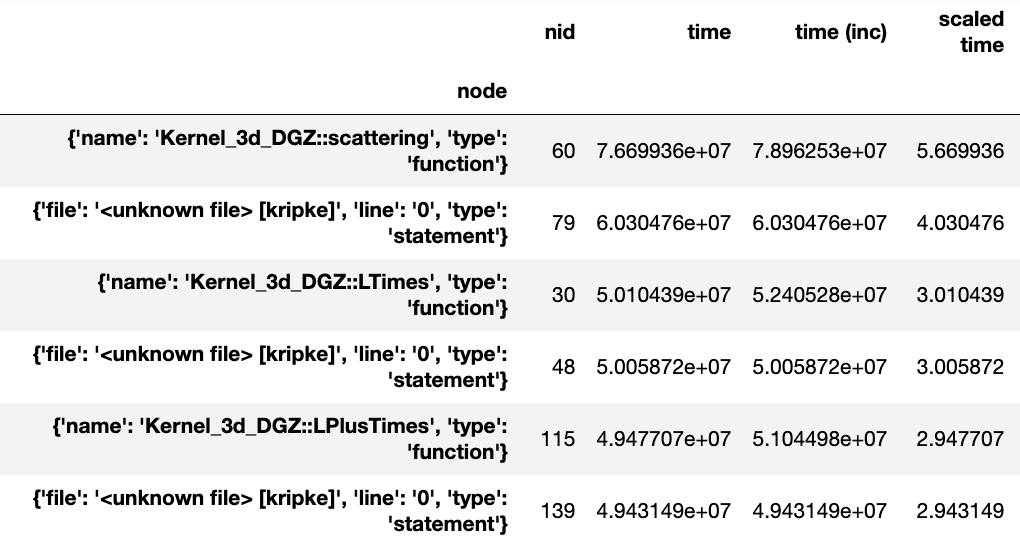### Generating a flat profile¶

We can generate a flat profile in hatchet by using the `groupby` functionality in pandas. The flat profile can be based on any categorical column (e.g., function name, load module, file name). We can transform the tree or graph generated by a profiler into a flat profile by specifying the column on which to apply the `groupby` operation and the function to use for aggregation.

In the example below, we apply a pandas `groupby` operation on the `name` column. The time spent in each function is computed using `sum` to aggregate rows in a group. We then display the resulting DataFrame sorted by time.

```# Read in Kripke HPCToolkit database.
gf = ht.GraphFrame.from_hpctoolkit('kripke')

# Drop all index levels in the DataFrame except ``node``.
gf.drop_index_levels()

# Group DataFrame by ``name`` column, compute sum of all rows in each
# group. This shows the aggregated time spent in each function.
grouped = gf.dataframe.groupby('name').sum()

# Sort DataFrame by ``time`` column in descending order.
sorted_df = grouped.sort_values(by=['time'],
ascending=False)

# Display resulting DataFrame.
print(sorted_df)
```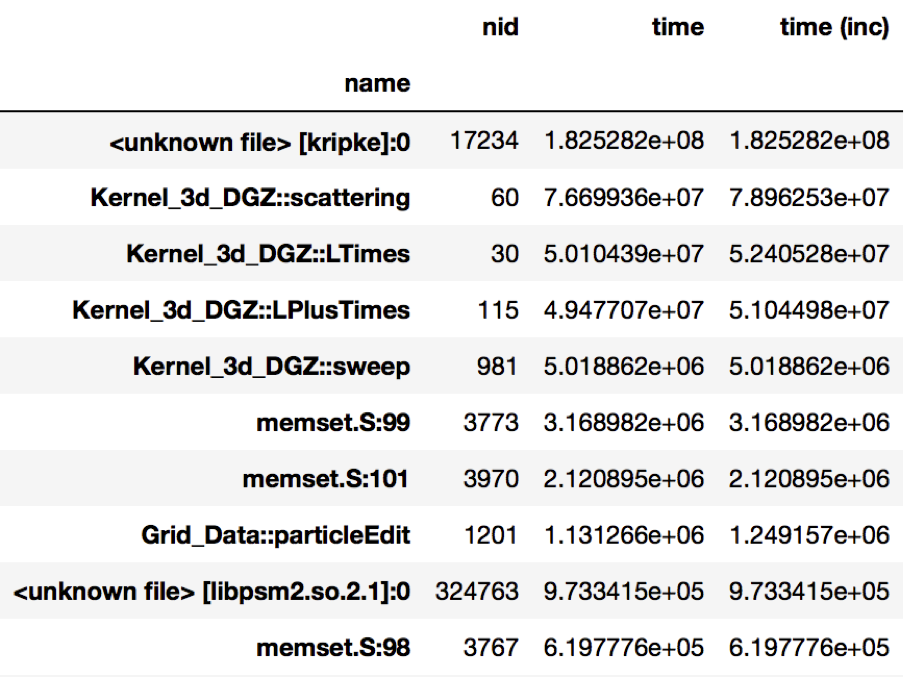Figure 1: Resulting DataFrame after performing a groupby on the `name` column in this HPCToolkit dataset (only showing a handful of rows for brevity). The DataFrame is sorted in descending order by the `time` column to show the function name with the biggest execution time.

### Identifying load imbalance¶

Hatchet makes it extremely easy to study load imbalance across processes or threads at the per-node granularity (call site or function level). A typical metric to measure imbalance is to look at the ratio of the maximum and average time spent in a code region across all processes.

In this example, we ran LULESH across 512 cores, and are interested in understanding the imbalance across processes. We first perform a `drop_index_levels` operation on the GraphFrame in two different ways: (1) by providing mean as a function in one case, and (2) max as the function to another copy of the DataFrame. This generates two DataFrames, one containing the average time spent in each node, and the other containing the maximum time spent in each node by any process. If we divide the corresponding columns of the two DataFrames and look at the nodes with the highest value of the max-to-average ratio, we can identify the nodes with highest imbalance.

```# Read in LULESH Caliper dataset.
gf1 = ht.GraphFrame.from_caliper('lulesh-512cores')

# Create a copy of the GraphFrame.
gf2 = gf1.copy()

# Drop all index levels in gf1's DataFrame except ``node``, computing the
# average time spent in each node.
gf1.drop_index_levels(function=np.mean)

# Drop all index levels in a copy of gf1's DataFrame except ``node``, this
# time computing the max time spent in each node.
gf2.drop_index_levels(function=np.max)

# Compute the imbalance by dividing the ``time`` column in the max DataFrame
# (i.e., gf2) by the average DataFrame (i.e., gf1). This creates a new column
# called ``imbalance`` in gf1's DataFrame.
gf1.dataframe['imbalance'] = gf2.dataframe['time'].div(gf1.dataframe['time'])

# Sort DataFrame by ``imbalance`` column in descending order.
sorted_df = gf1.dataframe.sort_values(by=['imbalance'], ascending=False)

# Display resulting DataFrame.
print(sorted_df)
```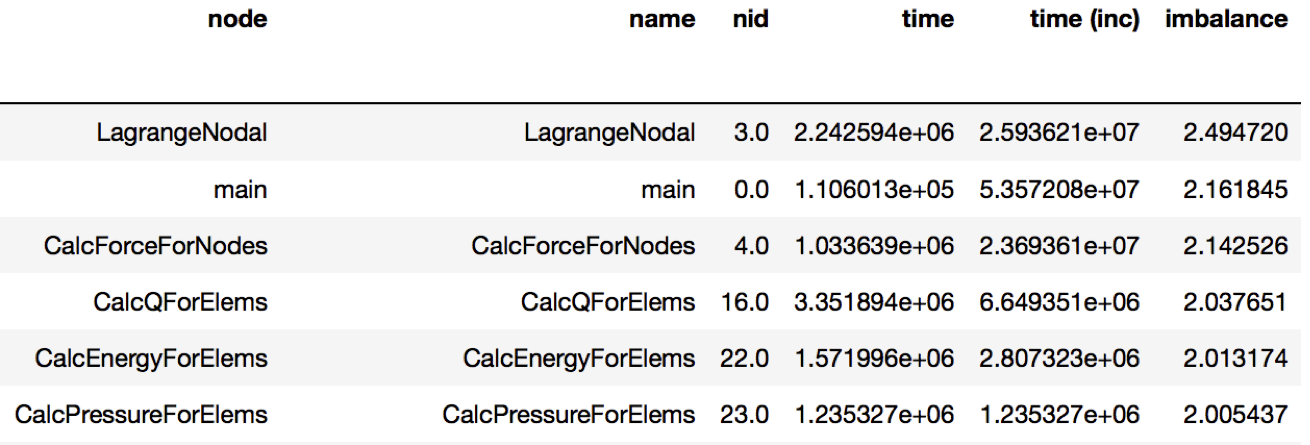Figure 2: Resulting DataFrame showing the imbalance in this Caliper dataset (only showing a handful of rows for brevity). The DataFrame is sorted in descending order by the new `imbalance` column calculated by dividing the max/average time of each function. The function with the highest level of imbalance within a node is `LagrangeNodal` with an imbalance of 2.49.

### Comparing multiple executions¶

An important task in parallel performance analysis is comparing the performance of an application on two different thread counts or process counts. The `filter`, `squash`, and `subtract` operations provided by the Hatchet API can be extremely powerful in comparing profiling datasets from two executions.

In the example below, we ran LULESH at two core counts: 1 core and 27 cores, and wanted to identify the performance changes as one scales on a node. We subtract the GraphFrame at 27 cores from the GraphFrame at 1 core (after dropping the additional index levels), and sort the resulting GraphFrame by execution time.

```# Read in LULESH Caliper dataset at 1 core.
gf1 = ht.GraphFrame.from_caliper('lulesh-1core.json')

# Read in LULESH Caliper dataset at 27 cores.
gf2 = ht.GraphFrame.from_caliper('lulesh-27cores.json')

# Drop all index levels in gf2's DataFrame except ``node``.
gf2.drop_index_levels()

# Subtract the GraphFrame at 27 cores from the GraphFrame at 1 core, and
# store result in a new GraphFrame.
gf3 = gf2 - gf1

# Sort resulting DataFrame by ``time`` column in descending order.
sorted_df = gf3.dataframe.sort_values(by=['time'], ascending=False)

# Display resulting DataFrame.
print(sorted_df)
```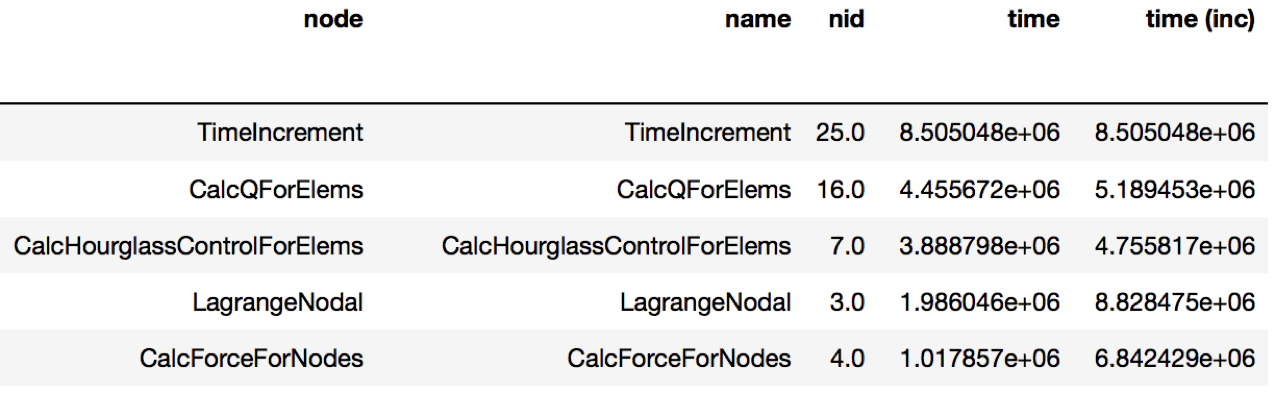Figure 3: Resulting DataFrame showing the performance differences when running LULESH at 1 core vs. 27 cores (only showing a handful of rows for brevity). The DataFrame sorts the function names in descending order by the `time` column. The `TimeIncrement` has the largest difference in execution time of 8.5e6 as the code scales from 1 to 27 cores.

### Filtering by library¶

Sometimes, users are interested in analyzing how a particular library, such as PetSc or MPI, is used by their application and how the time spent in the library changes as we scale to a larger number of processes.

In this next example, we compare two datasets generated from executions at different numbers of MPI processes. We read in two datasets of LULESH at 27 and 512 MPI processes, respectively, and filter them both on the `name` column by matching the names against `^MPI`. After the filtering operation, we `squash` the DataFrames to generate GraphFrames that just contain the MPI calls from the original datasets. We can now subtract the squashed datasets to identify the biggest offenders.

```# Read in LULESH Caliper dataset at 27 cores.
gf1 = GraphFrame.from_caliper('lulesh-27cores')

# Drop all index levels in DataFrame except ``node``.
gf1.drop_index_levels()

# Filter GraphFrame by names that start with ``MPI``. This only filters the #
# DataFrame. The Graph and DataFrame are now out of sync.
filtered_gf1 = gf1.filter(lambda x: x['name'].startswith('MPI'))

# Squash GraphFrame, the nodes in the Graph now match what's in the
# DataFrame.
squashed_gf1 = filtered_gf1.squash()

# Read in LULESH Caliper dataset at 512 cores, drop all index levels except
# ``node``, filter and squash the GraphFrame, leaving only nodes that start
# with ``MPI``.
gf2 = GraphFrame.from_caliper('lulesh-512cores')
gf2.drop_index_levels()
filtered_gf2 = gf2.filter(lambda x: x['name'].startswith('MPI'))
squashed_gf2 = filtered_gf2.squash()

# Subtract the two GraphFrames, store the result in a new GraphFrame.
diff_gf = squashed_gf2 - squashed_gf1

# Sort resulting DataFrame by ``time`` column in descending order.
sorted_df = diff_gf.dataframe.sort_values(by=['time'], ascending=False)

# Display resulting DataFrame.
print(sorted_df)
```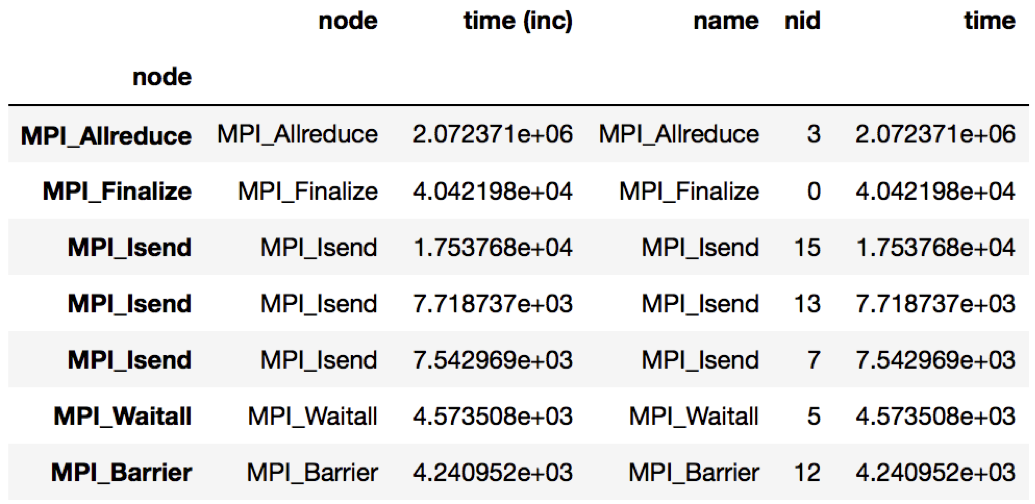Figure 4: Resulting DataFrame showing the MPI performance differences when running LULESH at 27 cores vs. 512 cores. The DataFrame sorts the MPI functions in descending order by the `time` column. In this example, the `MPI_Allreduce` function sees the largest increase in time scaling from 27 to 512 cores.

## Scaling Performance Examples¶

### Analyzing strong scaling performance¶

Hatchet can be used for a strong scaling analysis of applications. In this example, we compare the performance of LULESH running on 1 and 64 cores. By executing a simple `divide` of the two datasets in Hatchet, we can quickly pinpoint bottleneck functions. In the resulting graph, we invert the color scheme, so that functions that did not scale well (i.e., have a low speedup) are colored in red.

```gf_1core = ht.GraphFrame.from_caliper('lulesh*-1core.json')
gf_64cores = ht.GraphFrame.from_caliper('lulesh*-64cores.json')

gf_64cores["time"] *= 64

gf_strong_scale = gf_1core / gf_64cores
```

### Analyzing weak scaling performance¶

Hatchet can be used for comparing parallel scaling performance of applications. In this example, we compare the performance of LULESH running on 1 and 27 cores. By executing a simple `divide` of the two datasets in Hatchet, we can quickly identify which function calls did or did not scale well. In the resulting graph, we invert the color scheme, so that functions that did not scale well (i.e., have a low speedup) are colored in red.

```gf_1core = ht.GraphFrame.from_caliper('lulesh*-1core.json')
gf_27cores = ht.GraphFrame.from_caliper('lulesh*-27cores.json')

gf_weak_scale = gf_1core / gf_27cores
```

### Identifying scaling bottlenecks¶

Hatchet can also be used to analyze data in a weak or strong scaling performance study. In this example, we ran LULESH from 1 to 512 cores on third powers of some numbers. We read in all the datasets into Hatchet, and for each dataset, we use a few lines of code to filter the regions where the code spends most of the time. We then use the pandas’ pivot and plot operations to generate a stacked bar chart that shows how the time spent in different regions of LULESH changes as the code scales.

```# Grab all LULESH Caliper datasets, store in a sorted list.
datasets = glob.glob('lulesh*.json')
datasets.sort()

# For each dataset, create a new GraphFrame, and drop all index levels,
# except ``node``. Insert filtered graphframe into a list.
dataframes = []
for dataset in datasets:
gf = ht.GraphFrame.from_caliper(dataset)
gf.drop_index_levels()

# Grab the number of processes from the file name, store this as a new
# column in the DataFrame.
num_pes = re.match('(.*)-(\d+)(.*)', dataset).group(2)
gf.dataframe['pes'] = num_pes

# Filter the GraphFrame keeping only those rows with ``time`` greater
# than 1e6.
filtered_gf = gf.filter(lambda x: x['time'] > 1e6)

# Insert the filtered GraphFrame into a list.
dataframes.append(filtered_gf.dataframe)

# Concatenate all DataFrames into a single DataFrame called ``result``.
result = pd.concat(dataframes)

# Reshape the Dataframe, such that ``pes`` is an index column, ``name``
# fields are the new column names, and the values for each cell is the
# ``time`` fields.
pivot_df = result.pivot(index='pes', columns='name', values='time')

# Make a stacked bar chart using the data in the pivot table above.
pivot_df.loc[:,:].plot.bar(stacked=True, figsize=(10,7))
```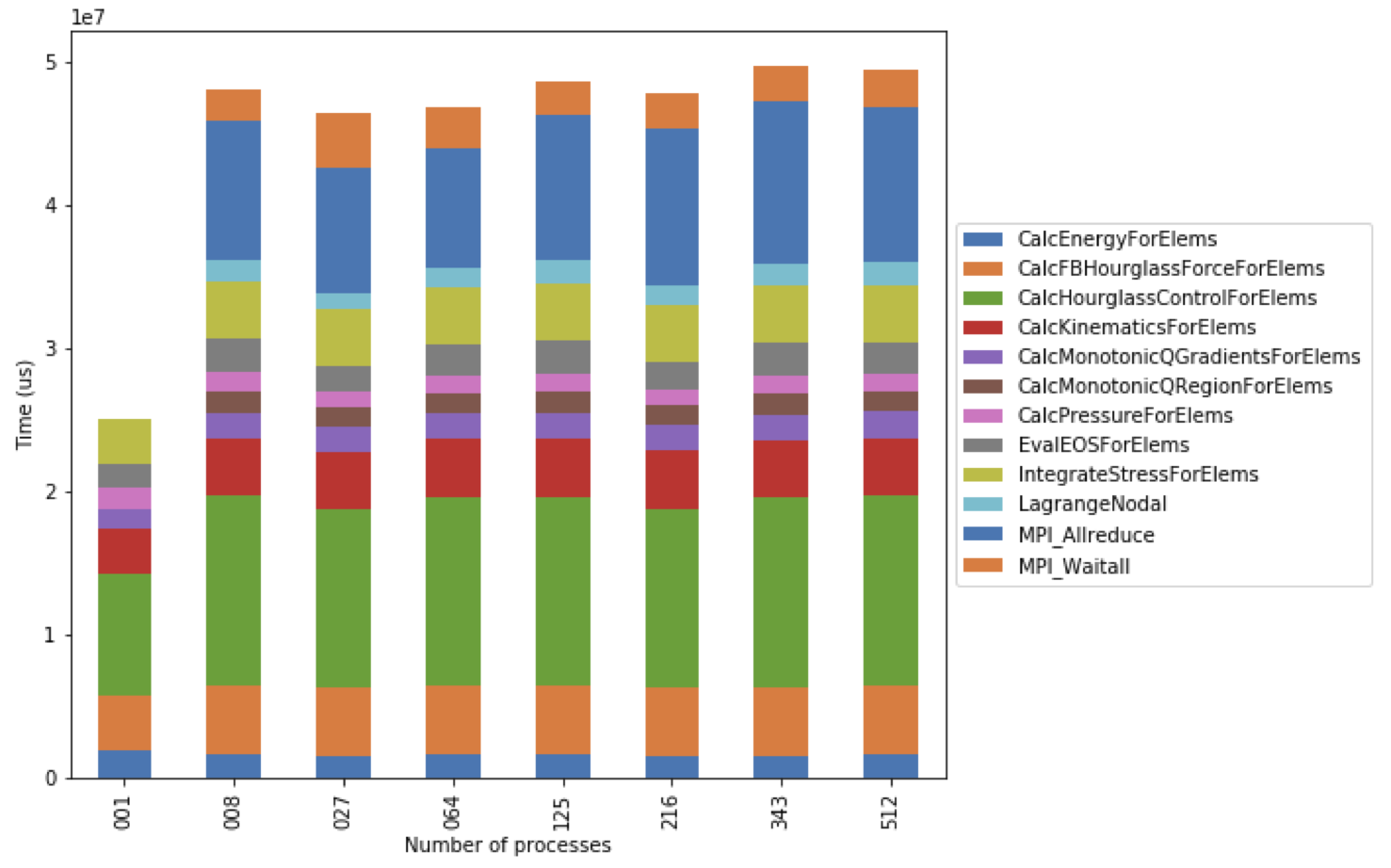Figure 5: Resulting stacked bar chart showing the time spent in different functions in LULESH as the code scales from 1 up to 512 processes. In this example, the `CalcHourglassControlForElems` function increases in runtime moving from 1 to 8 processes, then stays constant.

We use the same LULESH scaling datasets above to filter for time-consuming functions that start with the string `Calc`. This data is used to produce a line chart showing the performance of each function as the number of processes is increased. One of the functions (`CalcMonotonicQRegionForElems`) does not occur until the number of processes is greater than 1.

```datasets = glob.glob('lulesh*.json')
datasets.sort()

dataframes = []
for dataset in datasets:
gf = ht.GraphFrame.from_caliper(dataset)
gf.drop_index_levels()

num_pes = re.match('(.*)-(\d+)(.*)', dataset).group(2)
gf.dataframe['pes'] = num_pes
filtered_gf = gf.filter(lambda x: x["time"] > 1e6 and x["name"].startswith('Calc'))
dataframes.append(filtered_gf.dataframe)

result = pd.concat(dataframes)
pivot_df = result.pivot(index='pes', columns='name', values='time')
pivot_df.loc[:,:].plot.line(figsize=(10, 7))
```